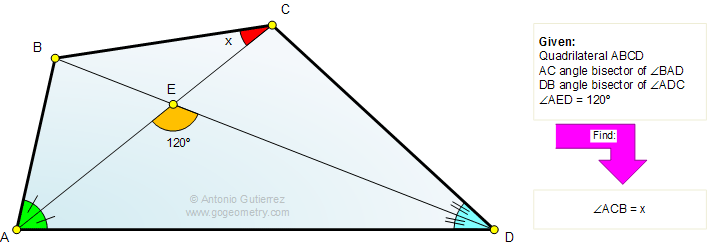Online Geometry Problem 698: Quadrilateral, Diagonal, Angle Bisector, Triangle, 120 Degrees. Level: High School, Honors Geometry, College, Mathematics Education

 The figure shows a quadrilateral ABCD. AC is the angle bisector of angle BAD, DB is the angle bisector of angle ADC. If the measure of angle AED is 120 degrees, find the measure of angle ACB. See also typography of problem 698.Home | Search | Geometry | Problems | All Problems | Open Problems | Visual Index | Problems Art Gallery | 691-700 | Triangles | Quadrilaterals | 120 degrees | Angle Bisector | Angles | Email | Post a comment or solution | By Antonio Gutierrez